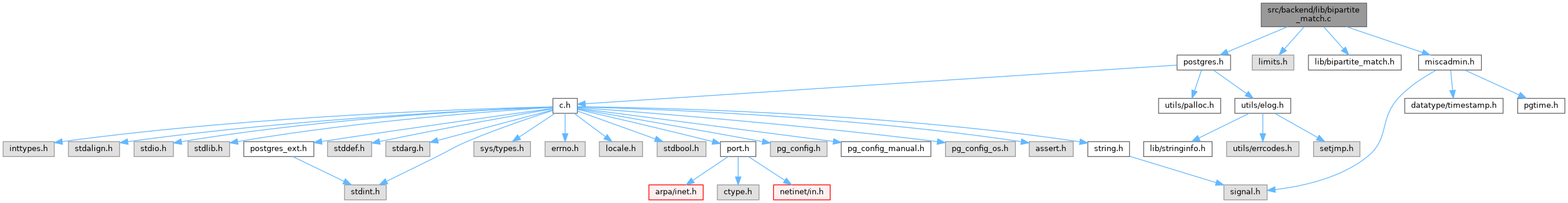PostgreSQL Source Code  git master
bipartite_match.c File Reference
`#include "postgres.h"`
`#include <limits.h>`
`#include "lib/bipartite_match.h"`
`#include "miscadmin.h"`
Include dependency graph for bipartite_match.c:Go to the source code of this file.

## Macros

#define HK_INFINITY   SHRT_MAX

## Functions

static bool hk_depth_search (BipartiteMatchState *state, int u)

BipartiteMatchStateBipartiteMatch (int u_size, int v_size, short **adjacency)

void BipartiteMatchFree (BipartiteMatchState *state)

## ◆ HK_INFINITY

 #define HK_INFINITY   SHRT_MAX

Definition at line 29 of file bipartite_match.c.

## ◆ BipartiteMatch()

 BipartiteMatchState* BipartiteMatch ( int u_size, int v_size, short ** adjacency )

Definition at line 39 of file bipartite_match.c.

40 {
42
43  if (u_size < 0 || u_size >= SHRT_MAX ||
44  v_size < 0 || v_size >= SHRT_MAX)
45  elog(ERROR, "invalid set size for BipartiteMatch");
46
47  state->u_size = u_size;
48  state->v_size = v_size;
50  state->matching = 0;
51  state->pair_uv = (short *) palloc0((u_size + 1) * sizeof(short));
52  state->pair_vu = (short *) palloc0((v_size + 1) * sizeof(short));
53  state->distance = (short *) palloc((u_size + 1) * sizeof(short));
54  state->queue = (short *) palloc((u_size + 2) * sizeof(short));
55
57  {
58  int u;
59
60  for (u = 1; u <= u_size; u++)
61  {
62  if (state->pair_uv[u] == 0)
63  if (hk_depth_search(state, u))
64  state->matching++;
65  }
66
67  CHECK_FOR_INTERRUPTS(); /* just in case */
68  }
69
70  return state;
71 }
static bool hk_depth_search(BipartiteMatchState *state, int u)
#define ERROR
Definition: elog.h:33
#define elog(elevel,...)
Definition: elog.h:218
void * palloc0(Size size)
Definition: mcxt.c:1093
void * palloc(Size size)
Definition: mcxt.c:1062
#define CHECK_FOR_INTERRUPTS()
Definition: regguts.h:318

References CHECK_FOR_INTERRUPTS, elog, ERROR, hk_breadth_search(), hk_depth_search(), palloc(), and palloc0().

Referenced by extract_rollup_sets().

## ◆ BipartiteMatchFree()

 void BipartiteMatchFree ( BipartiteMatchState * state )

Definition at line 78 of file bipartite_match.c.

79 {
80  /* adjacency matrix is treated as owned by the caller */
81  pfree(state->pair_uv);
82  pfree(state->pair_vu);
83  pfree(state->distance);
84  pfree(state->queue);
85  pfree(state);
86 }
void pfree(void *pointer)
Definition: mcxt.c:1169

References pfree().

Referenced by extract_rollup_sets().

 static bool hk_breadth_search ( BipartiteMatchState * state )
static

Definition at line 93 of file bipartite_match.c.

94 {
95  int usize = state->u_size;
96  short *queue = state->queue;
97  short *distance = state->distance;
98  int qhead = 0; /* we never enqueue any node more than once */
99  int qtail = 0; /* so don't have to worry about wrapping */
100  int u;
101
102  distance = HK_INFINITY;
103
104  for (u = 1; u <= usize; u++)
105  {
106  if (state->pair_uv[u] == 0)
107  {
108  distance[u] = 0;
110  }
111  else
112  distance[u] = HK_INFINITY;
113  }
114
116  {
117  u = queue[qtail++];
118
119  if (distance[u] < distance)
120  {
123
124  for (; i > 0; i--)
125  {
127
128  if (distance[u_next] == HK_INFINITY)
129  {
130  distance[u_next] = 1 + distance[u];
131  Assert(qhead < usize + 2);
133  }
134  }
135  }
136  }
137
138  return (distance != HK_INFINITY);
139 }
#define HK_INFINITY
int i
Definition: isn.c:73
Assert(fmt[strlen(fmt) - 1] !='\n')

References Assert(), HK_INFINITY, and i.

Referenced by BipartiteMatch().

## ◆ hk_depth_search()

 static bool hk_depth_search ( BipartiteMatchState * state, int u )
static

Definition at line 146 of file bipartite_match.c.

147 {
148  short *distance = state->distance;
149  short *pair_uv = state->pair_uv;
150  short *pair_vu = state->pair_vu;
153  short nextdist;
154
155  if (u == 0)
156  return true;
157  if (distance[u] == HK_INFINITY)
158  return false;
159  nextdist = distance[u] + 1;
160
162
163  for (; i > 0; i--)
164  {
166
167  if (distance[pair_vu[v]] == nextdist)
168  {
169  if (hk_depth_search(state, pair_vu[v]))
170  {
171  pair_vu[v] = u;
172  pair_uv[u] = v;
173  return true;
174  }
175  }
176  }
177
178  distance[u] = HK_INFINITY;
179  return false;
180 }
void check_stack_depth(void)
Definition: postgres.c:3470

References check_stack_depth(), HK_INFINITY, and i.

Referenced by BipartiteMatch().